# NCERT Solutions for Class 10th: Ch 8 Introduction to Trigonometry Maths

#### NCERT Solutions for Class 10th: Ch 8 Introduction to Trigonometry Maths

Excercise 8.1

Page No: 181

1. In Δ ABC, right-angled at B, AB = 24 cm, BC = 7 cm. Determine :
(i) sin A, cos A
(ii) sin C, cos C

In Δ ABC,∠B = 90º
By Applying Pythagoras theorem , we get
AC2 = AB2 + BC= (24)2 + 72 = (576+49) cm2 = 625 cm2
⇒ AC = 25
(i) sin A = BC/AC = 7/25    cos A = AB/AC = 24/25
(ii) sin C = AB/AC = 24/25
cos C = BC/AC = 7/25

2.  In Fig. 8.13, find tan P – cot R.

By Applying Pythagoras theorem in ΔPQR , we get
PR2 = PQ2 + QR2 = (13)2 = (12)2 + QR= 169 = 144  + QR2
⇒  QR2 = 25 ⇒  QR = 5 cm
Now,
tan P = QR/PQ = 5/12
cot R = QR/PQ = 5/12
A/q
tan P – cot R = 5/12 - 5/12 = 0

3. If sin A =3/4, calculate cos A and tan A.

Let ΔABC be a right-angled triangle, right-angled at B.
We know that sin A = BC/AC = 3/4
Let BC be 3k and AC will be 4k where k is a positive real number.
By Pythagoras theorem we get,
AC2 = AB2 + BC
(4k)2 = AB2 + (3k)2
16k2 - 9k2 = AB2
AB= 7k2
AB = √7 k
cos A = AB/AC = √7 k/4k = √7/4
tan A = BC/AB = 3k/7 k = 3/7

4. Given 15 cot A = 8, find sin A and sec A.

Let ΔABC be a right-angled triangle, right-angled at B.
We know that cot A = AB/BC = 8/15   (Given)
Let AB be 8k and BC will be 15k where k is a positive real number.
By Pythagoras theorem we get,
AC2 = AB2 + BC
AC2 = (8k)2 + (15k)2
AC2 = 64k2 + 225k2
AC2 = 289k2
AC = 17 k
sin A = BC/AC = 15k/17k = 15/17
sec A = AC/AB = 17k/8 k = 17/8

5. Given sec θ = 13/12, calculate all other trigonometric ratios.

Let ΔABC be a right-angled triangle, right-angled at B.
We know that sec θ = OP/OM = 13/12   (Given)
Let OP be 13k and OM will be 12k where k is a positive real number.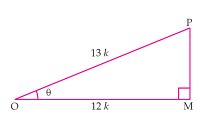By Pythagoras theorem we get,
OP2 = OM2 + MP
(13k)2 = (12k)+ MP
169k2 - 144k2 = MP2
MP2 = 25k2
MP = 5
Now,
sin θ = MP/OP = 5k/13k = 5/13
cos θ = OM/OP = 12k/13k = 12/13
tan θ = MP/OM = 5k/12k = 5/12
cot θ = OM/MP = 12k/5k = 12/5
cosec θ = OP/MP = 13k/5k = 13/5

6.  If ∠A and ∠B are acute angles such that cos A = cos B, then show that ∠A = ∠B.

A/q,
cos A = cos B
Let AD/BD = AC/BC = k
⇒ AD = kBD  .... (i)
⇒ AC = kBC  .... (ii)
By applying Pythagoras theorem in ΔCAD and ΔCBD we get,
CD2 = AC2 - AD2 …. (iii)
and also CD2 = BC2 - BD2 …. (iv)
From equations (iii) and (iv) we get,
AC2 - AD2 = BC2 - BD2
⇒ (kBC)2 - (k BD)2 = BC2 - BD2
⇒ k2 (BC2 - BD2) = BC2 - BD2
⇒ k2 = 1
⇒ k = 1
Putting this value in equation (ii), we obtain
AC = BC
⇒ ∠A = ∠B  (Angles opposite to equal sides of a triangle are equal-isosceles triangle)

7. If cot θ =7/8, evaluate :
(i)(1+sin θ )(1-sin θ)/(1+cos θ)(1-cos θ)
(ii) cot2θ

A/q,
cot θ = BC/AB = 7/8
Let BC = 7k and AB = 8k, where k is a positive real number.
By Pythagoras theorem in ΔABC we get.
AC2 = AB2 + BC
AC2 = (8k)2 + (7k)2
AC2 = 64k2 + 49k2
AC2 = 113k2
AC = √113 k
sin θ = AB/AC = 8k/113 k = 8/113
and cos θ = BC/AC = 7k/113 k = 7/113
(i) (1+sin θ )(1-sin θ)/(1+cos θ)(1-cos θ) = (1-sin2θ)/(1-cos2θ) = {1 - (8/113)2}/{1 - (7/113)2}
= {1 - (64/113)}/{1 - (49/113)} = {(113 - 64)/113}/{(113 - 49)/113} = 49/64
(ii) cot2θ = (7/8)2 = 49/64

8.  If 3cot A = 4/3 , check whether (1-tan2A)/(1+tan2A) = cos2A – sin2A or not.Let ΔABC in which ∠B = 90º,
A/q,
cot A = AB/BC = 4/3
Let AB = 4k and BC = 3k, where k is a positive real number.
By Pythagoras theorem in ΔABC we get.
AC2 = AB2 + BC
AC2 = (4k)2 + (3k)2
AC2 = 16k2 + 9k2
AC2 = 25k2
AC = 5k
tan A = BC/AB = 3/4
sin A = BC/AC = 3/5
cos A = AB/AC = 4/5
L.H.S. = (1-tan2A)/(1+tan2A) = 1- (3/4)2/1+ (3/4)= (1- 9/16)/(1+ 9/16) = (16-9)/(16+9) = 7/25
R.H.S. = cos2A – sin2A = (4/5)2 - (3/4)2 = (16/25) - (9/25) = 7/25
R.H.S. = L.H.S.
Hence,  (1-tan2A)/(1+tan2A) = cos2A – sin2A

9. In triangle ABC, right-angled at B, if tan A =1/3 find the value of:
(i) sin A cos C + cos A sin C
(ii) cos A cos C – sin A sin C

Let ΔABC in which ∠B = 90º,
A/q,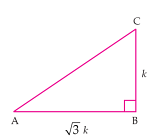tan A = BC/AB = 1/3
Let AB = √3 k and BC = k, where k is a positive real number.
By Pythagoras theorem in ΔABC we get.
AC2 = AB2 + BC
AC2 = (√3 k)2 + (k)2
AC2 = 3k2 + k2
AC2 = 4k2
AC = 2k
sin A = BC/AC = 1/2                   cos A = AB/AC = √3/2 ,
sin C = AB/AC = √3/2                   cos A = BC/AC = 1/2
(i) sin A cos C + cos A sin C = (1/2×1/2) + (√3/2×√3/2) = 1/4+3/4 = 4/4 = 1
(ii) cos A cos C – sin A sin C = (√3/2×1/2) - (1/2×√3/2) = √3/4 - √3/4 = 0

10. In Δ PQR, right-angled at Q, PR + QR = 25 cm and PQ = 5 cm. Determine the values of sin P, cos P and tan P.

Given that, PR + QR = 25 , PQ = 5
Let PR be x.  ∴ QR = 25 - x
By Pythagoras theorem ,
PR2 = PQ2 + QR2
x2 = (5)2 + (25 - x)2
x2 = 25 + 625 + x2 - 50x
50x = 650
x = 13
∴ PR = 13 cm
QR = (25 - 13) cm = 12 cm
sin P = QR/PR = 12/13
cos P = PQ/PR = 5/13
tan P = QR/PQ = 12/5

11.  State whether the following are true or false. Justify your answer.
(i) The value of tan A is always less than 1.
(ii) sec A = 12/5 for some value of angle A.
(iii) cos A is the abbreviation used for the cosecant of angle A.
(iv) cot A is the product of cot and A.
(v) sin θ = 4/3 for some angle θ.

In ΔABC in which ∠B = 90º,
AB = 3, BC = 4 and AC = 5
Value of tan A = 4/3 which is greater than.
The triangle can be formed with sides equal to 3, 4 and hypotenuse = 5 as
it will follow the Pythagoras theorem.AC2 = AB2 + BC
52 = 32 + 42
25 = 9 + 16
25 = 25

(ii) True.
Let a ΔABC in which ∠B = 90º,AC be 12k and AB be 5k, where k is a positive real number.
By Pythagoras theorem we get,
AC2 = AB2 + BC
(12k)2 = (5k)2 + BC
BC+ 25k= 144k2
BC= 119k2
Such a triangle is possible as it will follow the Pythagoras theorem.

(iii) False.
Abbreviation used for cosecant of angle A is cosec A.cos A is the abbreviation used for cosine of angle A.

(iv) False.
cot A is not the product of cot and A. It is the cotangent of ∠A.

(v) False.
sin θ = Height/Hypotenuse
We know that in a right angled triangle, Hypotenuse is the longest side.
∴ sin θ will always less than 1 and it can never be 4/3 for any value of θ.

Excercise 8.2

Page No: 187

1. Evaluate the following :
(i) sin 60° cos 30° + sin 30° cos 60° (ii) 2 tan245° + cos230° – sin260°
(iii) cos 45°/(sec 30° + cosec 30°)    (iv) (sin 30° + tan 45° – cosec 60°)/(sec 30° + cos 60° + cot 45°)
(v) (5cos260° + 4sec230° - tan245°)/(sin230° + cos230°)

(i) sin 60° cos 30° + sin 30° cos 60°
=  (√3/2×√3/2) + (1/2×1/2) = 3/4 + 1/4 = 4/4 = 1

(ii) 2 tan245° + cos230° – sin260°
= 2×(1)+ (√3/2)2 - (√3/2)= 2

(iii) cos 45°/(sec 30° + cosec 30°)
= 1/√2/(2/√3 + 2) = 1/√2/{(2+2√3)/3)
= √3/2×(2+2√3) = √3/(2√2+2√6)
= √3(2√6-2√2)/(2√6+2√2)(2√6-2√2)
= 2√3(√6-√2)/(2√6)2-(2√2)2
=  2√3(√6-√2)/(24-8) =  2√3(√6-√2)/16
= √3(√6-√2)/8 = (√18-√6)/8 = (3√2-√6)/8

(iv) (sin 30° + tan 45° – cosec 60°)/(sec 30° + cos 60° + cot 45°)
= (1/2+1-2/√3)/(2/√3+1/2+1)
= (3/2-2/√3)/(3/2+2/√3)
= (3√3-4/2√3)/(3√3+4/2√3)
= (3√3-4)/(3√3+4)
= (3√3-4)(3√3-4)/(3√3+4)(3√3-4)
= (3√3-4)2/(3√3)2-(4)2
= (27+16-24√3)/(27-16)
= (43-24√3)/11]

(v) (5cos260° + 4sec230° - tan245°)/(sin230° + cos230°)
= 5(1/2)2+4(2/√3)2-12/(1/2)2+(√3/2)2
= (5/4+16/3-1)/(1/4+3/4)
= (15+64-12)/12/(4/4)
= 67/12

2. Choose the correct option and justify your choice :
(i) 2tan 30°/1+tan230° =
(A) sin 60°            (B) cos 60°          (C) tan 60°            (D) sin 30°
(ii) 1-tan245°/1+tan245° =
(A) tan 90°            (B) 1                    (C) sin 45°            (D) 0
(iii)  sin 2A = 2 sin A is true when A =
(A) 0°                   (B) 30°                  (C) 45°                 (D) 60°
(iv) 2tan30°/1-tan230° =
(A) cos 60°          (B) sin 60°             (C) tan 60°           (D) sin 30°

(i) (A) is correct.
2tan 30°/1+tan230° = 2(1/√3)/1+(1/√3)2
= (2/√3)/(1+1/3) = (2/√3)/(4/3)
= 6/4√3 = √3/2 = sin 60°

(ii)  (D) is correct.
1-tan245°/1+tan245° = (1-12)/(1+12)
= 0/2 = 0

(iii) (A) is correct.
sin 2A = 2 sin A is true when A =
= As sin 2A = sin 0° = 0
2 sin A = 2sin 0° = 2×0 = 0
or,
sin 2A = 2sin A cos A
⇒2sin A cos A = 2 sin A
⇒ 2cos A = 2 ⇒ cos A = 1
⇒ A = 0°

(iv) (C) is correct.
2tan30°/1-tan230° =  2(1/√3)/1-(1/√3)2
= (2/√3)/(1-1/3) = (2/√3)/(2/3) = √3 = tan 60°

3. If tan (A + B) = √3 and tan (A – B) = 1/3; 0° < A + B ≤ 90°; A > B, find A and B.

tan (A + B) = √3
⇒ tan (A + B) = tan 60°
⇒ (A + B) = 60° ... (i)
tan (A – B) = 1/3
⇒ tan (A - B) = tan 30°
⇒ (A - B) = 30° ... (ii)
Adding (i) and (ii), we get
A + B + A - B = 60° + 30°
2A = 90°
A= 45°
Putting the value of A in equation (i)
45° + B = 60°
⇒ B = 60° - 45°
⇒ B = 15°
Thus, A = 45° and B = 15°

4. State whether the following are true or false. Justify your answer.
(i) sin (A + B) = sin A + sin B.
(ii) The value of sin θ increases as θ increases.
(iii) The value of cos θ increases as θ increases.
(iv) sin θ = cos θ for all values of θ.
(v) cot A is not defined for A = 0°.

(i) False.
Let A = 30° and B = 60°, then
sin (A + B) = sin (30° + 60°) = sin 90° = 1 and,
sin A + sin B = sin 30° + sin 60°
= 1/2 + √3/2 = 1+√3/2

(ii) True.
sin 0° = 0
sin 30° = 1/2
sin 45° = 1/2
sin 60° = √3/2
sin  90° = 1
Thus the value of sin θ increases as θ increases.

(iii) False.
cos 0° = 1
cos 30° = √3/2
cos 45° = 1/2
cos 60° = 1/2
cos 90° = 0
Thus the value of cos θ decreases as θ increases.

(iv) True.
cot A = cos A/sin A
cot 0° = cos 0°/sin 0° = 1/0 = undefined.

Excercise 8.3

Page No : 189

1. Evaluate :
(i) sin 18°/cos 72°        (ii) tan 26°/cot 64°        (iii)  cos 48° – sin 42°       (iv)  cosec 31° – sec 59°

(i) sin 18°/cos 72°
= sin (90° - 18°) /cos 72°
= cos 72° /cos 72° = 1

(ii) tan 26°/cot 64°
= tan (90° - 36°)/cot 64°
= cot 64°/cot 64° = 1

(iii) cos 48° - sin 42°
= cos (90° - 42°) - sin 42°
= sin 42° - sin 42° = 0

(iv) cosec 31° - sec 59°
= cosec (90° - 59°) - sec 59°
= sec 59° - sec 59° = 0

2.  Show that :
(i) tan 48° tan 23° tan 42° tan 67° = 1
(ii) cos 38° cos 52° – sin 38° sin 52° = 0

(i) tan 48° tan 23° tan 42° tan 67°
= tan (90° - 42°) tan (90° - 67°) tan 42° tan 67°
= cot 42° cot 67° tan 42° tan 67°
= (cot 42° tan 42°) (cot 67° tan 67°) = 1×1 = 1

(ii) cos 38° cos 52° - sin 38° sin 52°
= cos (90° - 52°) cos (90°-38°) - sin 38° sin 52°
= sin 52° sin 38° - sin 38° sin 52° = 0

3. If tan 2A = cot (A – 18°), where 2A is an acute angle, find the value of A.

A/q,
tan 2A = cot (A- 18°)
⇒ cot (90° - 2A) = cot (A -18°)
Equating angles,
⇒ 90° - 2A = A- 18° ⇒ 108° = 3A
⇒ A = 36°

4.  If tan A = cot B, prove that A + B = 90°.

A/q,
tan A = cot B
⇒ tan A = tan (90° - B)
⇒ A = 90° - B
⇒ A + B = 90°

5. If sec 4A = cosec (A – 20°), where 4A is an acute angle, find the value of A.

A/q,
sec 4A = cosec (A - 20°)
⇒ cosec (90° - 4A) = cosec (A - 20°)
Equating angles,
90° - 4A= A- 20°
⇒ 110° = 5A
⇒ A = 22°

Page No : 190

6. If A, B and C are interior angles of a triangle ABC, then show that
sin (B+C/2) = cos A/2

In a triangle, sum of all the interior angles
A + B + C = 180°
⇒ B + C = 180° - A
⇒ (B+C)/2 = (180°-A)/2
⇒ (B+C)/2 = (90°-A/2)
⇒ sin (B+C)/2 = sin (90°-A/2)
⇒ sin (B+C)/2 = cos A/2

7. Express sin 67° + cos 75° in terms of trigonometric ratios of angles between 0° and 45°.

sin 67° + cos 75°
= sin (90° - 23°) + cos (90° - 15°)
= cos 23° + sin 15°

Excercise 8.4

Page No : 193

1. Express the trigonometric ratios sin A, sec A and tan A in terms of cot A.

cosec2A - cot2A = 1
⇒ cosec2A = 1 + cot2A
⇒ 1/sin2A = 1 + cot2A
⇒sin2A = 1/(1+cot2A)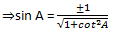Now,
sin2A = 1/(1+cot2A)
⇒ 1 - cos2A = 1/(1+cot2A)
⇒cos2A = 1 - 1/(1+cot2A)
⇒cos2A = (1-1+cot2A)/(1+cot2A)
⇒ 1/sec2A = cot2A/(1+cot2A)
⇒ secA = (1+cot2A)/cot2Aalso,
tan A = sin A/cos A and cot A = cos A/sin A
⇒ tan A = 1/cot A

2. Write all the other trigonometric ratios of ∠A in terms of sec A.

We know that,
sec A = 1/cos A
⇒ cos A = 1/sec A
also,
cos2A + sin2A = 1
⇒  sin2A = 1 - cos2A
⇒  sin2A = 1 - (1/sec2A)
⇒  sin2A = (sec2A-1)/sec2A

also,
sin A = 1/cosec A
⇒cosec A = 1/sin A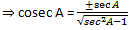Now,
sec2A - tan2A = 1
⇒ tan2A = sec2A + 1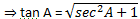also,
tan A = 1/cot A
⇒ cot A = 1/tan A3. Evaluate :
(i) (sin263° + sin227°)/(cos217° + cos273°)
(ii)  sin 25° cos 65° + cos 25° sin 65°

(i) (sin263° + sin227°)/(cos217° + cos273°)
= [sin2(90°-27°) + sin227°]/[cos2(90°-73°) + cos273°)]
= (cos227° + sin227°)/(sin227° + cos273°)
= 1/1 =1                       (∵ sin2A + cos2A = 1)

(ii) sin 25° cos 65° + cos 25° sin 65°
= sin(90°-25°) cos 65° + cos(90°-65°) sin 65°
= cos 65° cos 65° + sin 65° sin 65°
= cos265° + sin265° = 1

4. Choose the correct option. Justify your choice.
(i) 9 sec2A - 9 tan2A =
(A) 1                 (B) 9              (C) 8                (D) 0
(ii) (1 + tan θ + sec θ) (1 + cot θ - cosec θ)
(A) 0                 (B) 1              (C) 2                (D) - 1
(iii) (secA + tanA) (1 - sinA) =
(A) secA           (B) sinA        (C) cosecA      (D) cosA
(iv) 1+tan2A/1+cot2A =
(A) sec2A                 (B) -1              (C) cot2A                (D) tan2A

(i) (B) is correct.
9 sec2A - 9 tan2A
= 9 (sec2A - tan2A)
= 9×1 = 9             (∵ sec2 A - tan2 A = 1)

(ii) (C) is correct
(1 + tan θ + sec θ) (1 + cot θ - cosec θ)
= (1 + sin θ/cos θ + 1/cos θ) (1 + cos θ/sin θ - 1/sin θ)
= (cos θ+sin θ+1)/cos θ × (sin θ+cos θ-1)/sin θ
= (cos θ+sin θ)2-12/(cos θ sin θ)
= (cos2θ + sin2θ + 2cos θ sin θ -1)/(cos θ sin θ)
= (1+ 2cos θ sin θ -1)/(cos θ sin θ)
= (2cos θ sin θ)/(cos θ sin θ) = 2

(iii) (D) is correct.
(secA + tanA) (1 - sinA)
= (1/cos A + sin A/cos A) (1 - sinA)
= (1+sin A/cos A) (1 - sinA)
= (1 - sin2A)/cos A
= cos2A/cos A = cos A

(iv) (D) is correct.
1+tan2A/1+cot2
= (1+1/cot2A)/1+cot2A
= (cot2A+1/cot2A)×(1/1+cot2A)
= 1/cot2A = tan2A

5. Prove the following identities, where the angles involved are acute angles for which the
expressions are defined.
(i) (cosec θ - cot θ)= (1-cos θ)/(1+cos θ)
(ii) cos A/(1+sin A) + (1+sin A)/cos A = 2 sec A
(iii) tan θ/(1-cot θ) + cot θ/(1-tan θ) = 1 + sec θ cosec θ
[Hint : Write the expression in terms of sin θ and cos θ]
(iv) (1 + sec A)/sec A = sin2A/(1-cos A)
[Hint : Simplify LHS and RHS separately]
(v) (cos A–sin A+1)/(cos A+sin A–1) = cosec A + cot A,using the identity cosec2A = 1+cot2A.

(vii) (sin θ - 2sin3θ)/(2cos3θ-cos θ) = tan θ
(viii) (sin A + cosec A)+ (cos A + sec A)2 = 7+tan2A+cot2A
(ix) (cosec A – sin A)(sec A – cos A) = 1/(tan A+cotA)
[Hint : Simplify LHS and RHS separately]
(x) (1+tan2A/1+cot2A) = (1-tan A/1-cot A)2 = tan2A

(i) (cosec θ - cot θ)= (1-cos θ)/(1+cos θ)
L.H.S. =  (cosec θ - cot θ)2
= (cosec2θ + cot2θ - 2cosec θ cot θ)
= (1/sin2θ + cos2θ/sin2θ - 2cos θ/sin2θ)
= (1 + cos2θ - 2cos θ)/(1 - cos2θ)
= (1-cos θ)2/(1 - cosθ)(1+cos θ)
= (1-cos θ)/(1+cos θ) = R.H.S.

(ii)  cos A/(1+sin A) + (1+sin A)/cos A = 2 sec A
L.H.S. = cos A/(1+sin A) + (1+sin A)/cos A
= [cos2A + (1+sin A)2]/(1+sin A)cos A
= (cos2A + sin2A + 1 + 2sin A)/(1+sin A)cos A
= (1 + 1 + 2sin A)/(1+sin A)cos A
= (2+ 2sin A)/(1+sin A)cos A
= 2(1+sin A)/(1+sin A)cos A
= 2/cos A = 2 sec A = R.H.S.

(iii) tan θ/(1-cot θ) + cot θ/(1-tan θ) = 1 + sec θ cosec θ
L.H.S. = tan θ/(1-cot θ) + cot θ/(1-tan θ)
= [(sin θ/cos θ)/1-(cos θ/sin θ)] + [(cos θ/sin θ)/1-(sin θ/cos θ)]
= [(sin θ/cos θ)/(sin θ-cos θ)/sin θ] + [(cos θ/sin θ)/(cos θ-sin θ)/cos θ]
= sin2θ/[cos θ(sin θ-cos θ)] + cos2θ/[sin θ(cos θ-sin θ)]
= sin2θ/[cos θ(sin θ-cos θ)] - cos2θ/[sin θ(sin θ-cos θ)]
= 1/(sin θ-cos θ) [(sin2θ/cos θ) - (cos2θ/sin θ)]
= 1/(sin θ-cos θ) × [(sin3θ - cos3θ)/sin θ cos θ]
= [(sin θ-cos θ)(sin2θ+cos2θ+sin θ cos θ)]/[(sin θ-cos θ)sin θ cos θ]
= (1 + sin θ cos θ)/sin θ cos θ
= 1/sin θ cos θ + 1
= 1 + sec θ cosec θ = R.H.S.

(iv)  (1 + sec A)/sec A = sin2A/(1-cos A)
L.H.S. = (1 + sec A)/sec A
= (1 + 1/cos A)/1/cos A
= (cos A + 1)/cos A/1/cos A
= cos A + 1
R.H.S. = sin2A/(1-cos A)
= (1 - cos2A)/(1-cos A)
= (1-cos A)(1+cos A)/(1-cos A)
= cos A + 1
L.H.S. = R.H.S.

(v) (cos A–sin A+1)/(cos A+sin A–1) = cosec A + cot A,using the identity cosec2A = 1+cot2A.
L.H.S. = (cos A–sin A+1)/(cos A+sin A–1)
Dividing Numerator and Denominator by sin A,
= (cos A–sin A+1)/sin A/(cos A+sin A–1)/sin A
= (cot A - 1 + cosec A)/(cot A+ 1 – cosec A)
= (cot A - cosec2A + cot2A + cosec A)/(cot A+ 1 – cosec A) (using cosec2A - cot2A = 1)
= [(cot A + cosec A) - (cosec2A - cot2A)]/(cot A+ 1 – cosec A)
= [(cot A + cosec A) - (cosec A + cot A)(cosec A - cot A)]/(1 – cosec A + cot A)
=  (cot A + cosec A)(1 – cosec A + cot A)/(1 – cosec A + cot A)
=  cot A + cosec A = R.H.S.Dividing Numerator and Denominator of L.H.S. by cos A,= sec A + tan A = R.H.S.

(vii) (sin θ - 2sin3θ)/(2cos3θ-cos θ) = tan θ
L.H.S. = (sin θ - 2sin3θ)/(2cos3θ - cos θ)
= [sin θ(1 - 2sin2θ)]/[cos θ(2cos2θ- 1)]
= sin θ[1 - 2(1-cos2θ)]/[cos θ(2cos2θ -1)]
= [sin θ(2cos2θ -1)]/[cos θ(2cos2θ -1)]
= tan θ = R.H.S.

(viii) (sin A + cosec A)+ (cos A + sec A)2 = 7+tan2A+cot2A
L.H.S. = (sin A + cosec A)+ (cos A + sec A)2
= (sin2A + cosec2A + 2 sin A cosec A) + (cos2A + sec2A + 2 cos A sec A)
= (sin2A + cos2A) + 2 sin A(1/sin A) + 2 cos A(1/cos A) + 1 + tan2A + 1 + cot2A
= 1 + 2 + 2 + 2 + tan2A + cot2A
= 7+tan2A+cot2A = R.H.S.

(ix) (cosec A – sin A)(sec A – cos A) = 1/(tan A+cotA)
L.H.S. = (cosec A – sin A)(sec A – cos A)
= (1/sin A - sin A)(1/cos A - cos A)
= [(1-sin2A)/sin A][(1-cos2A)/cos A]
= (cos2A/sin A)×(sin2A/cos A)
= cos A sin A
R.H.S. = 1/(tan A+cotA)
= 1/(sin A/cos A +cos A/sin A)
= 1/[(sin2A+cos2A)/sin A cos A]
= cos A sin A
L.H.S. = R.H.S.

(x)  (1+tan2A/1+cot2A) = (1-tan A/1-cot A)2 = tan2A
L.H.S. = (1+tan2A/1+cot2A)
= (1+tan2A/1+1/tan2A)
= 1+tan2A/[(1+tan2A)/tan2A]
= tan2A